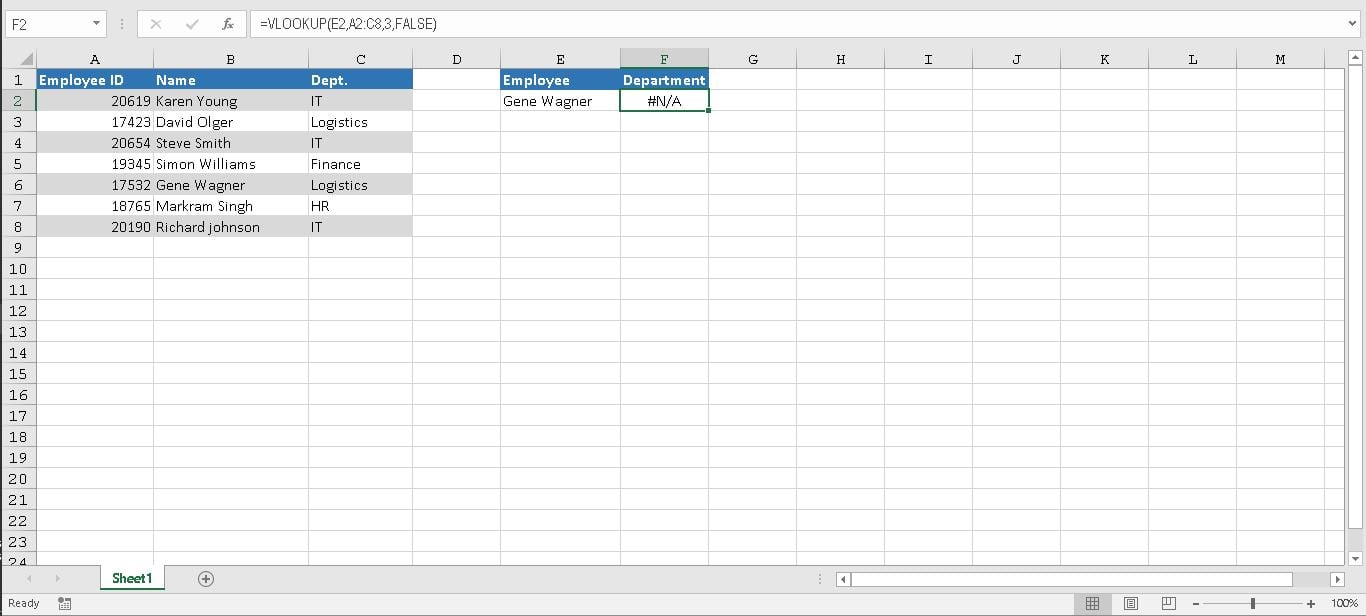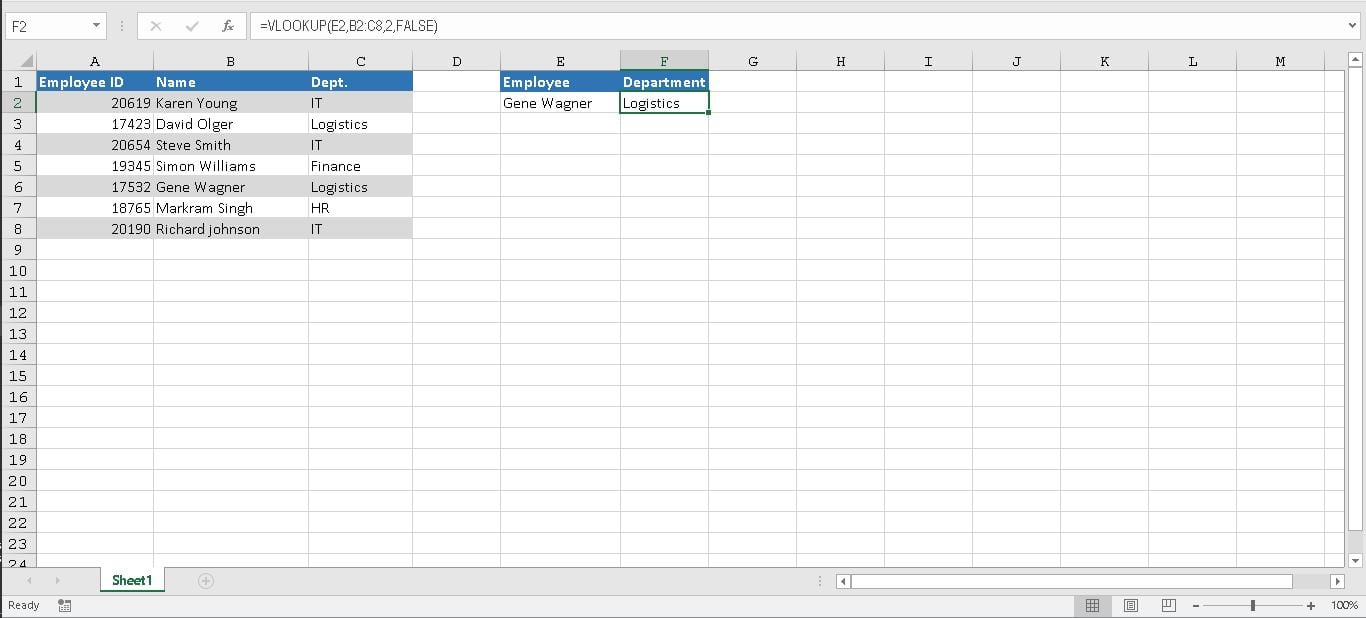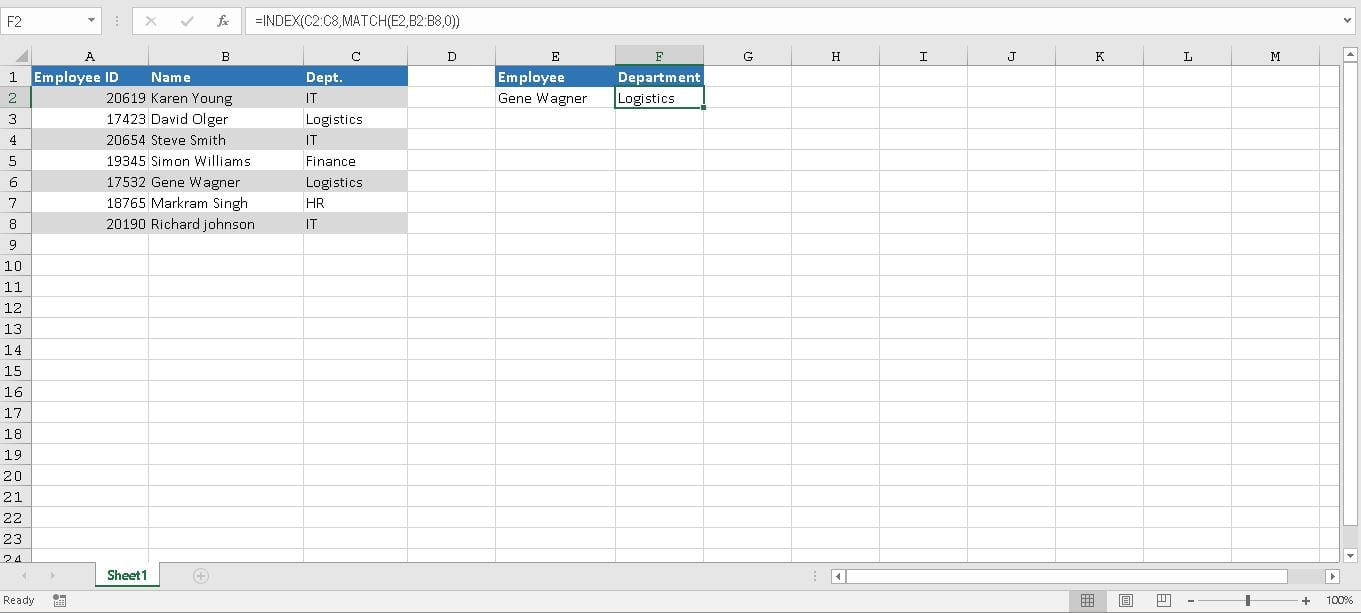Get instant live expert help with Excel or Google Sheets“My Excelchat expert helped me in less than 20 minutes, saving me what would have been 5 hours of work!”

#### Post your problem and you’ll get expert help in seconds.

Your message must be at least 40 characters
Our professional experts are available now. Your privacy is guaranteed.

# Find the Matching Value When the Lookup Value Is Not on the First Column of the Table Array

VLOOKUP is one of the lookup and reference functions in Excel is used to find values in a specified range by “row”. It compares them row-wise until it finds a match. In order for VLOOKUP to work, the lookup value must be on the left-most column on the table array. If your lookup value is not on the first column of the array, you will see the #N/A error. In this tutorial, we will look at some workarounds when the value is not in the first column.

### Using VLOOKUP for matches when the lookup value isn’t in the first column

We will use the employee records dataset for demonstration. Here we have the employee information on cells A2:C8. For each employee, we have their Employee ID, Name and departments. In the following table, we want to retrieve the department for “Gene Wagner”(E2) on cell F2. The VLOOKUP Formula in use is

`=VLOOKUP(E2,A2:C8,3,FALSE)`The #N/A error results because the lookup value “Gene Wagner” appears in the second column (Name) of the table array argument A2:C8. In this case, Excel is looking for it in column A, not column B.

The #N/A error can be resolved using one of the following fixes.

### Changing the table array reference

You can fix the #N/A error adjusting your VLOOKUP to refer the correct column. If that’s not possible, try to move the columns. But that might be impractical when you have a large dataset or maybe there are other logical reasons why you can’t move the columns. We can set the table array to B2:C8 which makes our formula

`=VLOOKUP(E2,B2:C8,2,FALSE)`### Using the CHOOSE function with VLOOKUP in an Array formula

You can use VLOOKUP with the CHOOSE function to create an Array formula which would look up E2 in B2:B8 and return a result from C2:C8 if successful. You would need to apply this formula using CTRL + SHIFT + ENTER. The formula will be

`=VLOOKUP(E2,CHOOSE({1,2},B2:B8, C2:C8),2,0)`### Limitations of the VLOOKUP function

There are certain limitations using VLOOKUP. Besides the lookup value in the first column, VLOOKUP needs the return a value in the specified range. You have to know the column number containing the return value. This can be very inefficient when you have a large table.

VLOOKUP makes a static reference to the table, so adding a new column to the table breaks the VLOOKUP formula. VLOOKUP will only look for a closest or exact match to a value. It also assumes by default that the first column in the table array is sorted alphabetically.

If your table is not sorted that way, VLOOKUP will return the first closest match which in many cases may not be your desired output. For these reasons, the best alternative for a VLOOKUP is a combination of INDEX/ MATCH functions.

### Using a combination of INDEX and MATCH functions

Using a combination INDEX and MATCH, we can find a matching value when the lookup value is not on the first column of the table array. INDEX() returns the value of a cell in a table based on the column and row number. MATCH() returns the position of a cell in a row or column.

Combining these two functions we can look up a value both horizontally and vertically. The combination of INDEX and MATCH overcomes the limitations of VLOOKUP. INDEX and MATCH don’t require the return value to be in the same column as the lookup column. You can specify either a row or a column as an array- or specify both. The lookup value doesn’t have to be on the first column with INDEX and MATCH; it can be anywhere.

INDEX and MATCH offer the flexibility of making dynamic reference to the column which contains the return value; we can add columns to your table without breaking the formula. INDEX and MATCH offer more options for matches. You can specify an exact, greater than or lesser than match.Back to our employee records example. To implement the combination of INDEX and MATCH, we will assign the formula `=INDEX(C2:C8,MATCH(E2,B2:B8,0)) `to cell F2. This will return the Department for “Gene Wagner” which is “Logistics.”

Finding matching value in your own data set can be more complex, and you might need to use techniques other than those listed above. If you are in a rush and want your problem answered by an Excel expert, try our service. The experts are available to help you 24/7 at the link to the right. The first question is free.

### Did this post not answer your question? Get a solution from connecting with the expert.Another blog reader asked this question today on Excelchat:
Solution examplesI have a file with two sheets. On the 1st, I have dollars in O13and in O16 a number signifying 1-6 people. On the second workbook is a matrix that corresponds to the dollar amount AND the number of people. For example, sheet 1 O13 is \$6,000. O16 is 3. I want a formula to find \$6,000 (not higher) on sheet 2 in column A. Then I want it to compare sheet 1 O16 to the correct column (B-G representing 1-6) on sheet 2 for an exact match to place in sheet1 O17. The range of sheet1 O13 is referenced on sheet2 A5-A383, and sheet 1 O16 is referenced on sheet2 B5:G383. I have an INDEX Match but it isn't quite working, yet.
Solved by B. B. in 60 minsI need to find an INDEX function that will convert the Call Day (a number 1-7) to the actual weekday found in row 1 of the DayofWeek named range. I don't know if I'm supposed to use the MATCH function as well or not.
Solved by D. D. in 11 minshelp with INDEX(IndexArea,MATCH(\$M\$5,MatchRows,0),MATCH(\$M\$6,MatchColumns,0))
Solved by Z. Y. in 12 minsI'm having trouble with an index & match formula where I'm looking up two different pieces of info in order to return a specific value.
Solved by K. D. in 54 minsHello, I have a big spreadsheet in which I need to know how many patients came in each month based on provider. I am using the following formula but I still get the N/A error. =INDEX('No Show Appts Data'!D:E, MATCH(1, 'No Show Appts Data'!D:D='No Shows Data'!M2)*('No Shows Data'!E:E='No Shows Data'!N1),0)) No Show Appts Data is the name of the sheet where Column D is Month Year of date in question and Column E is the provider. No Shows Data is the sheet where I am making all the formula calculation where Column M is Month and Year and Column N is the provider in question and therefore M2 is the month in question and N1 the provider in question. How do I fix this error? Thanks
Solved by F. H. in 40 mins## Subscribe to Excelchat.coAnother blog reader asked this question today on Excelchat: